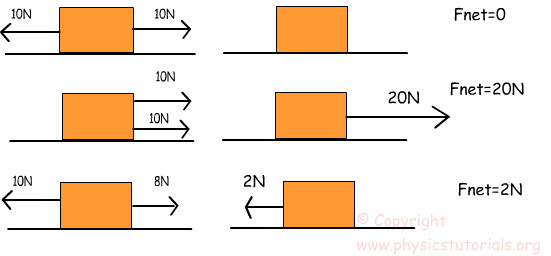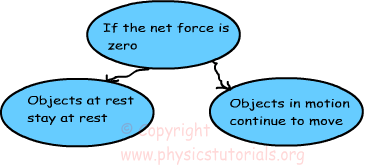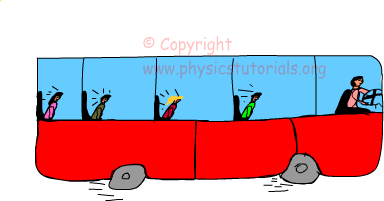# Newton's First Laws Of Motion

Newton’s First Law of Motion: In his first law of motion Newton stated that all objects save their state of motion. In other words, if an object is at rest it continuous to be at rest and if it is moving it continuous to move unless a nonzero force is applied on it. What we mean by a word “nonzero force”? Look at the given picture to understand what we mean.We called nonzero force “net force” also. As you see from the picture, if the forces acting on an object are in the same direction they are summed, If they are opposite direction we take one of them in negative direction and make calculations considering their signs and find resultant force vector. In first situation, the applied forces are opposite direction and since their magnitudes are same the net force becomes zero. In second situation, forces are in the same direction and they are also same in magnitude thus the resultant vector is the sum of them. And in the final situation forces are in the opposite direction, however, their magnitudes are different, so the resultant vector or net force has the direction of the force having larger magnitude.

After these explanations, I think net force concept is clear in your mind. Now let’s turn to our main topic “Newton’s first law of motion” in other words “law of inertia”. We said that, objects want to continue their state of motion whether they are at rest or in motion. If there is no net force on an object at rest than it continues to be at rest, if there is no net force on movingobject, it continues to move at constant speed. Look at the given picture below. As you see, passengers move forward when the driver breaks the bus. Passengers before the brake have same velocity with bus. Thus, because of the Newton’s first law, they tendto move with same speed. However, a sudden brake which is the result of a net force make passengers move forward. This is a good example for law of inertia from daily life. We can increase the number of example. For example you all experience the change in your body when in an accelerating elevator or at a swing.

Related